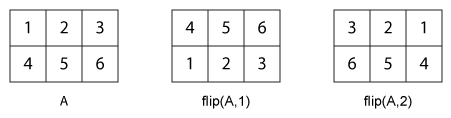# flip

Flip order of elements

## Syntax

``B = flip(A)``
``B = flip(A,dim)``

## Description

example

````B = flip(A)` returns array `B` the same size as `A`, but with the order of the elements reversed. The dimension that is reordered in `B` depends on the shape of `A`:If `A` is vector, then `flip(A)` reverses the order of the elements along the length of the vector.If `A` is a matrix, then `flip(A)` reverses the elements in each column.If `A` is an N-D array, then `flip(A)` operates on the first dimension of `A` in which the size value is not `1`.```

example

````B = flip(A,dim)` reverses the order of the elements in `A` along dimension `dim`. For example, if `A` is a matrix, then `flip(A,1)` reverses the elements in each column, and `flip(A,2)` reverses the elements in each row.```

## Examples

collapse all

```A = 'no word, no bond, row on.'; B = flip(A)```
```B = '.no wor ,dnob on ,drow on' ```
```A = [1;2;3]; B = flip(A)```
```B = 3×1 3 2 1 ```

Create a diagonal matrix, `A`.

`A = diag([100 200 300])`
```A = 3×3 100 0 0 0 200 0 0 0 300 ```

Flip `A` without specifying the `dim` argument.

`B = flip(A)`
```B = 3×3 0 0 300 0 200 0 100 0 0 ```

Now, flip `A` along the second dimension.

`B = flip(A,2)`
```B = 3×3 0 0 100 0 200 0 300 0 0 ```

Create a 1-by-3-by-2 array.

```A = zeros(1,3,2); A(:,:,1) = [1 2 3]; A(:,:,2) = [4 5 6]; A```
```A = A(:,:,1) = 1 2 3 A(:,:,2) = 4 5 6 ```

Flip `A` without specifying the `dim` argument.

`B = flip(A)`
```B = B(:,:,1) = 3 2 1 B(:,:,2) = 6 5 4 ```

Now, flip `A` along the third dimension.

`B = flip(A,3)`
```B = B(:,:,1) = 4 5 6 B(:,:,2) = 1 2 3 ```

Create a 3-by-2 cell array.

`A = {'foo',1000; 999,true; 'aaa','bbb'}`
```A=3×2 cell array {'foo'} {} {} {[ 1]} {'aaa'} {'bbb' } ```

Flip `A` without specifying the `dim` argument.

`B = flip(A)`
```B=3×2 cell array {'aaa'} {'bbb' } {} {[ 1]} {'foo'} {} ```

Now, flip `A` along the second dimension.

`B = flip(A,2)`
```B=3×2 cell array {} {'foo'} {[ 1]} {} {'bbb' } {'aaa'} ```

## Input Arguments

collapse all

Input array, specified as a vector, matrix, multidimensional array, table, or timetable.

Data Types: `double` | `single` | `int8` | `int16` | `int32` | `int64` | `uint8` | `uint16` | `uint32` | `uint64` | `logical` | `char` | `string` | `struct` | `cell` | `table` | `timetable` | `categorical` | `datetime` | `duration` | `calendarDuration`

Example: `[1 2 3 4]`

Example: `['abcde']`

Example: `[1 2; 3 4]`

Example: ```{'abcde',[1 2 3]}```

Example: `table(rand(1,5),rand(1,5))`

Dimension to operate along, specified as a positive integer scalar. If you do not specify the dimension, then the default is the first array dimension of size greater than 1.

The following illustration shows the difference between `dim=1` and `dim=2` when `A` is a matrix.## Version History

Introduced in R2013b